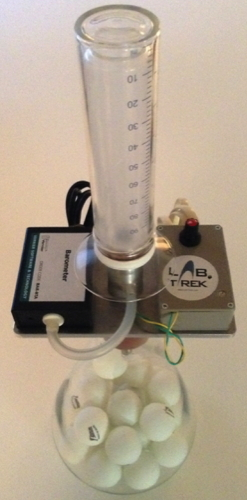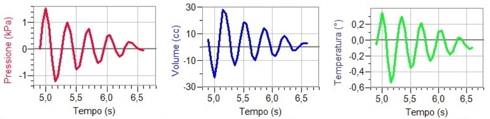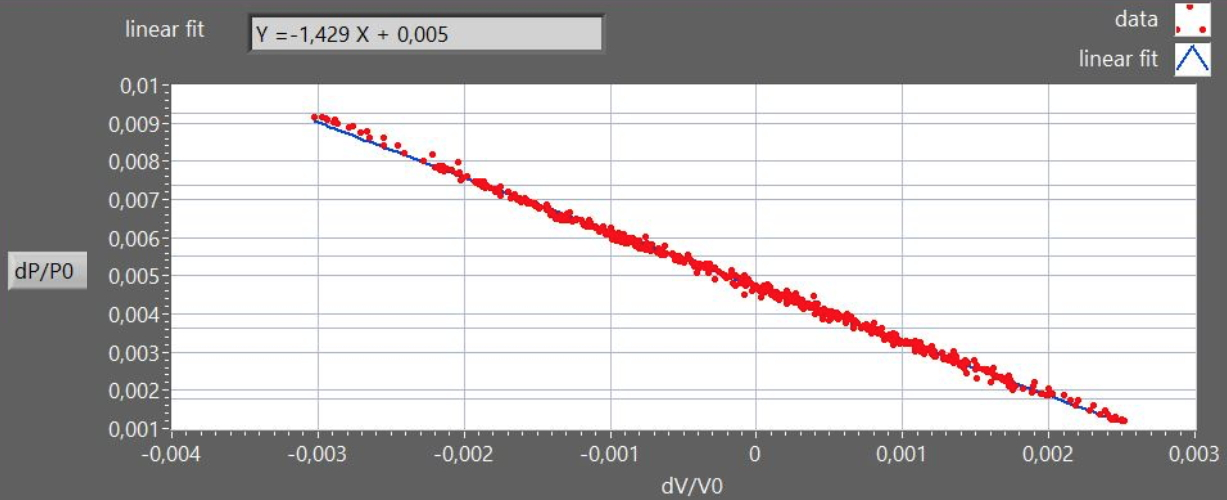Rüchardt's experiment for measuring γ=cp/cv in gasesThe apparatus (figure 1) allows to measure the ratio γ=Cp/Cv for air, using a modern version of the Rüchardt method, where Cp=specific heat at constant pressure and Cv=specific heat at constant volume. With this apparatus one simultaneously measures the changes of three thermodynamic variables (pressure, volume and temperature) during the fast volume oscillations induced in a mass of air by a piston sliding with low friction (adiabatic transformation). Volume is measured by a motion sensor (sonar), pressure is measured by a pressure sensor (barometer),  temperature is measured by a special sensor that monitors the resistance of a  tungsten wire . In adiabatic regime, where the Poisson relation holds (PVγ=cost), the γ value may be obtained not only, as in classic Rüchardt method, from the measured oscillation period, but also from direct interpolation of the P-V plot. For small changes in pressure  dP and volume dV the Poisson relation is dP= -(γP/V)dV A small displacement x of the piston from the equilibrium position, produces a volume change dV = xA (where A is the piston cross section) and a restoring force :  F =A dP = - A2 x (γ P/V). The free piston oscillations are therefore a (damped) harmonic motion (figure 2). A plot (figure 3) of the reduced variables dP/P0 vs. dV/V0, is a straight line with slope - γ. The dynamic may be varied by changing the value of the oscillating mass m (by loading the piston with metal rings) or the effective air volume V (by inserting ping-pong balls into the vessel ). see: A new MBLversion of the Rüchardt experiment Am. J. Phys. 69, (2001)Figure 1Figure 2Figure 3